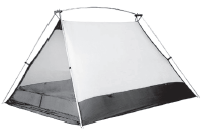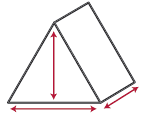# Geometric figures

A geometric figure is any combination of points, lines, or planes. Geometric figures are often classified as space figure, plane figure, lines, line segments, rays, and points depending on the dimensions of the figure.

## Space figure

A space figure is a three-dimensional geometric figure, or a figure that has length, width and height. It often occupies space and has volume when the figure is closed.

Example

The tent shown below is in the shape of a triangular prism. The drawing depicts the three dimensions of a triangular prism.## Plane figure

A plane figure is a two-dimensional geometric figure.It has no thickness and lies entirely in one plane.

Example

The triangular prism shown below has plane figures for faces. Two of these faces are triangles.

## Lines, line segments, and rays

Lines, line segments, and rays are one-dimensional geometric figures.

Example

The base of this triangle is a line segment. It has one dimension, length:

## Points

In the triangle above, the vertices are labeled A, B, and C. These points could be called zero-dimensional geometric figures because they do not occupy space.

See also line, line segment, 1D, plane figure, point, ray, space figure, 3D, 2D, 0D.